By clicking “Accept”, you agree to the storing of cookies on your device to enhance site navigation, analyze site usage, and assist in our marketing efforts. View our Privacy Policy for more information.

# Trader Simulation Explained (1/3)

This article covers an enhanced realization of trading activity simulation derived from the principles described previously and presents in detail the process behind choosing the best distributions for describing different traders, as well as proposes a way to generalize the estimated distribution parameters.

This allows us to model distinct market behaviors and present the main insights from conducting the simulations.

# Traders Simulation Summary

The examined use-case pairs are composed of non-traded security (X) and stablecoin (Y). The primary assets taken into consideration are private equity and real estate assets.

The stream of trades comes from a random process that “draws” trades from distributions. As in the case of non-traded securities, there are no alternative markets that would stimulate arbitrage activity in case of significant price differences.

Thus, it’s reasonable to assume that the behavior of traders for each side (those willing to exchange the security token for stablecoin and vice-versa) can be described by separate distributions, varying the parameters of which it would be possible to model distinct trading behaviors.

The main parameters needed to describe the behavior of traders for each side are:

• ✅ Trade Frequency – drawn from a Poisson distribution with parameter Lambda (λ – is the expected rate of occurrences every minute)
• ✅ Trade Size –  the distribution allowing to describe the traders best is to be determined

# Identification of Best-Fit Trade Size Distribution

The identification of the best-fit trade size distribution is based on the analysis of the historical Uniswap v2 transactions. Particularly, the proposed method is to focus primarily on the transactions of exchanging the stablecoin for some other token.

By determining the best-fit distribution for each pool containing the stablecoin on one side, it would be possible to generalize the results in order to highlight several distinct trading behaviors (which could be described by the parameters of the distribution).

It was determined that the focus would initially only be on the stablecoin swap in transactions because the values of exchanging an alternative token are directly linked to its price, which would significantly complicate the process of comparing traders’ patterns across distinct pools.

Another important aspect to consider is the liquidity inside the pool at the moment of performing the swap. When the liquidity is low, the traders are less likely to execute bigger transactions because of the significant price impact they cause.

To illustrate the point, let’s consider a simple example. In the case of a pool with Tokens A and B, with underlying reserves of 500:500 and disregarding the insignificant fee for liquidity providers, the amount of Token B a user would receive trying to swap 10 units of Token A is about 9.8 (exchange rate ~ 0.98).

Now, consider the case where the user tries to exchange 100 units of Token A instead. This time, the amount of Token B he would receive would only be 83.33 (exchange rate ~ 0.83, which is much worse than for the smaller swap). However, if the initial liquidity would be 100 times higher (50,000:50,000), the amount of Token B received by the user in the second transaction would be significantly higher – about 99.8.

Therefore, considering that in low liquidity pools the exchange rate is much worse for the users,  it makes sense to fit the transaction values to distributions separately, considering the liquidity before executing the transaction.

Selected reserve ranges (for stablecoin):

• 0 – 1 000
• 1,000 – 10,000
• 10,000 – 50,000
• 50,000 – 100,000
• 100,000 – 200,000
• 200,000 – 500,000
• 500,000 – 1,000,000
• 1,000,000 – 10,000,000
• 10,000,000 – 1,000,000,000

To categorize the transactions into corresponding reserve ranges, one of the options would be to look at the exact values of the reserves right before the transaction was executed. However, this method would fail for swaps ‘sandwiched’ by MEV-bot transactions and is susceptible to other manipulatory scenarios.

Another approach would be to consider the daily reserve values. The problem that arises now is the misclassification of transactions in case of significant mints or burns during the day, which would change the liquidity from one range to another.

In this case, the transactions executed before the significant variation in reserves would be classified into the wrong range (in case only the end of day reserves are considered). To avoid such scenarios, we consider only the transactions for which the end of day stablecoin reserves value didn’t deviate by more than 30% compared to the previous day’s value.

# Considered Distributions

In the analysis below, the distribution of swap-in stablecoin values (across each analyzed pool and for each corresponding reserve range separately) are compared against four (4) distinct distributions: LogNormal, Gamma, Weibull, HalfCauchy.

To compare how well each of these distributions fit the data, three (3) metrics have been chosen: SSE, MAE, and AIC.

SSE (error sum of squares) and MAE (mean absolute error) are computed based on the difference between the normalized histogram of the sample data and the PDF (probability density function) of the distribution estimated from the sample data using the maximum likelihood estimation method. The number of bins at which to compute the error is selected in order to have a bin width of 1,000, but cannot be less than 20 (number of bins = max(20, max(amount_in_values) / 1,000).

AIC (Akaike Information Criterion) is another effective method for choosing the best-fit distribution that deals with the trade-off between the goodness of fit of the model and its simplicity. The problem that it addresses is the tendency to overfit the more complex models. The metric is computed based on the likelihood of the examined distribution fitted using the MLE method and the number of estimated parameters (which serves as a penalty to avoid overfitting).

Compared to the previous two (2) metrics, the absolute value of AIC is meaningless (as it’s data specific) and can be used only to compare models fitted on identical samples. The smaller the AIC value, the better the model describing the data.

For all compared distributions, in order to estimate the parameters, the location parameter was fixed to 0 (reflecting the fact that the swap amount should take positive values) and the remaining parameters have been computed using the maximum likelihood estimation method.

Presented below is the table of errors calculated based on the stablecoin swaps from the pool WBTC/DAI during reserve that ranges from 10,000-50,000 and 50,000-100,000.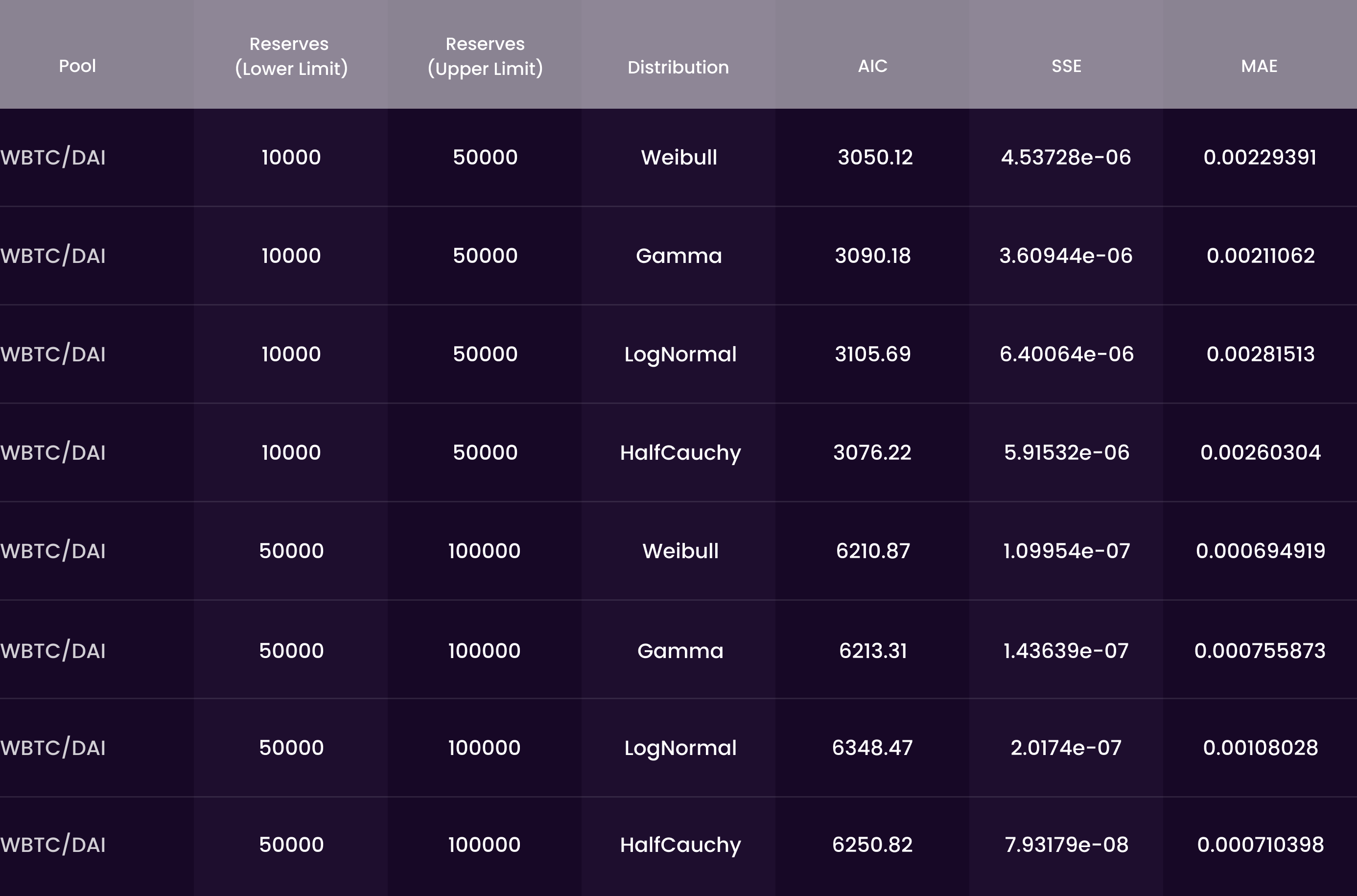Figure 1. Table of Errors, Pool: WBTC/DAI, Reserve Ranges: [10,000-50 000] and [50,000-100,000], Metrics: AIC (smaller-better), SSE (smaller-better), MAE (smaller-better)

The Weibull distribution shows the best performance according to AIC for both reserves ranges and outperforms the other distributions according to the MAE metric in the second reserve range. Gamma distribution has a better performance according to SSE and MAE metrics in the first reserve range.

Considering all analyzed reserve ranges across the examined pools, the final table of scores has been computed for each distribution. The score represents the number of cases in the distribution that outperformed the other ones according to the given metric.

The Weibull distribution has the best fit in the majority of the cases according to all examined metrics. The second best distribution is Gamma. Lognormal outperforms HalfCauchy based on AIC core but shows a poorer score based on MAE and SSE.

# Visual Methods

The examined metrics represent an effective way for model selection and filtering out bad-fit distributions, but they don’t provide any additional information about where and how much the fitted distributions deviate from the real data. To address this issue, visual methods can be applied.

One of the ways to assess visually the quality of fit is to overlay the distribution PDF on the histogram of the data.

From the plot above, it seems that all of the selected distributions provide a really good approximation for the trade-size data, with the Weibull distribution having a slightly better performance. However, there are several major downsides of the applied visual method for comparing similar distribution types.

First, the histogram of the real data depends too much on the number of bins. Particularly, if the number of bins would be increased, it would be visible that there is a decrease in the number of transactions having a very small amount, which is associated with high gas fees, that discourages users from performing very small trades.

Now, these trades are being binned with the ones that are slightly greater and extremely frequent and the mentioned aspect is being hidden. Also, it is not much clear what happens in the tail. As the probability of high values is very small for all selected distributions, it’s hard to identify the differences between them.

A more effective way to determine visually whether the data follows a particular distribution is using Q-Q plots (quantile-quantile plots). To construct the graph, theoretical quantiles (on the x-axis) are plotted against sample quantiles (on the y-axis). If the data follows a straight line, the distribution is considered to fit the data well.

In the Q-Q plots above, the points represent how well each historical swap in value compares to the value that would be generated using the given distribution. In case the points lie above the straight line, it means that the values generated in the given range (OX axis) are too small compared to the historical data (OY axis).

In contrast, if the points lie below the line, it means that the values are just too large. Slight deviations are acceptable, but in case they are too big and frequent, it signals that the given distribution describes the data poorly.

It can be seen that both Weibull and Gamma distributions provide a really good fit to the data. For the LogNormal distribution, starting from a given value, all of the generated points are much bigger compared to the historical value (notice the maximum sample value, which represents the historical data, is 10,000 while the theoretical generated maximum value is about 20,000). The HalfCauchy distribution, having a very fat tail, has the worst fit.

As Weibull distribution showed the best performance according to all of the considered metrics and provides a good visual fit in the majority of the cases, it was decided to proceed further with Weibull distribution for trade-size distribution parameter estimation and generalization.

# Weibull Distribution

The Weibull distribution is a continuous probability distribution used extensively in a lot of distinct fields due to its flexibility to model different shapes. The two-parameter Weibull distribution is described by the shape and scale parameters.

By increasing the scale parameter, the distribution is being stretched to the right. The shape parameter, unsurprisingly, determines the shape of the distribution. A smaller shape indicates a larger amount of extreme values and increasing the shape parameter leads to a shift of the distribution mode to the right and a consequent decrease of the tail thickness.

Previously, the fit of the distribution was assessed visually using Q-Q plots. One of the main downsides of applying Q-Q plots in order to assess the quality of fit for a heavy-tailed distribution is that the higher values (which represent only a small proportion of data) take up the majority of the space from the graph, with the main concentration of points being squeezed in the bottom-left corner.

The Weibull probability plots, which are constructed on the log-scaled x-axis, and y-axis scaled according to a special formula, address this issue.

To construct the probability plot, the historical data for each pool/reserve range combination (swap sizes) are sorted, scaled logarithmically, and plotted on the x-axis. The y-axis represents the quantiles of the Weibull distribution, converted into probability values.

# Elimination of Bad-Fit Pools

Even though Weibull distribution models the best trade-size in most of the analyzed pools, there are cases in which it has a poor performance. Before proceeding to generalize the parameters, it’s important to filter out the cases with a bad fit, so that they wouldn’t screw up the real picture.

Considering that on the Weibull Probability Plot, the points should ideally lie on a straight line, one of the ways to measure the quality of the fit is to compute the correlation coefficient. A value close to 1 suggests that the data follows the specified distribution. For the final estimations, the cases with a correlation coefficient below 0.95 have been filtered out.

# Estimated Parameters

In order to visualize the estimated parameters and understand how they vary as the liquidity changes, let’s construct a curve for each pool, with X-axis indicating the upper limit of the reserve range used for parameter estimation and the Y-axis indicating the value of the estimated parameter.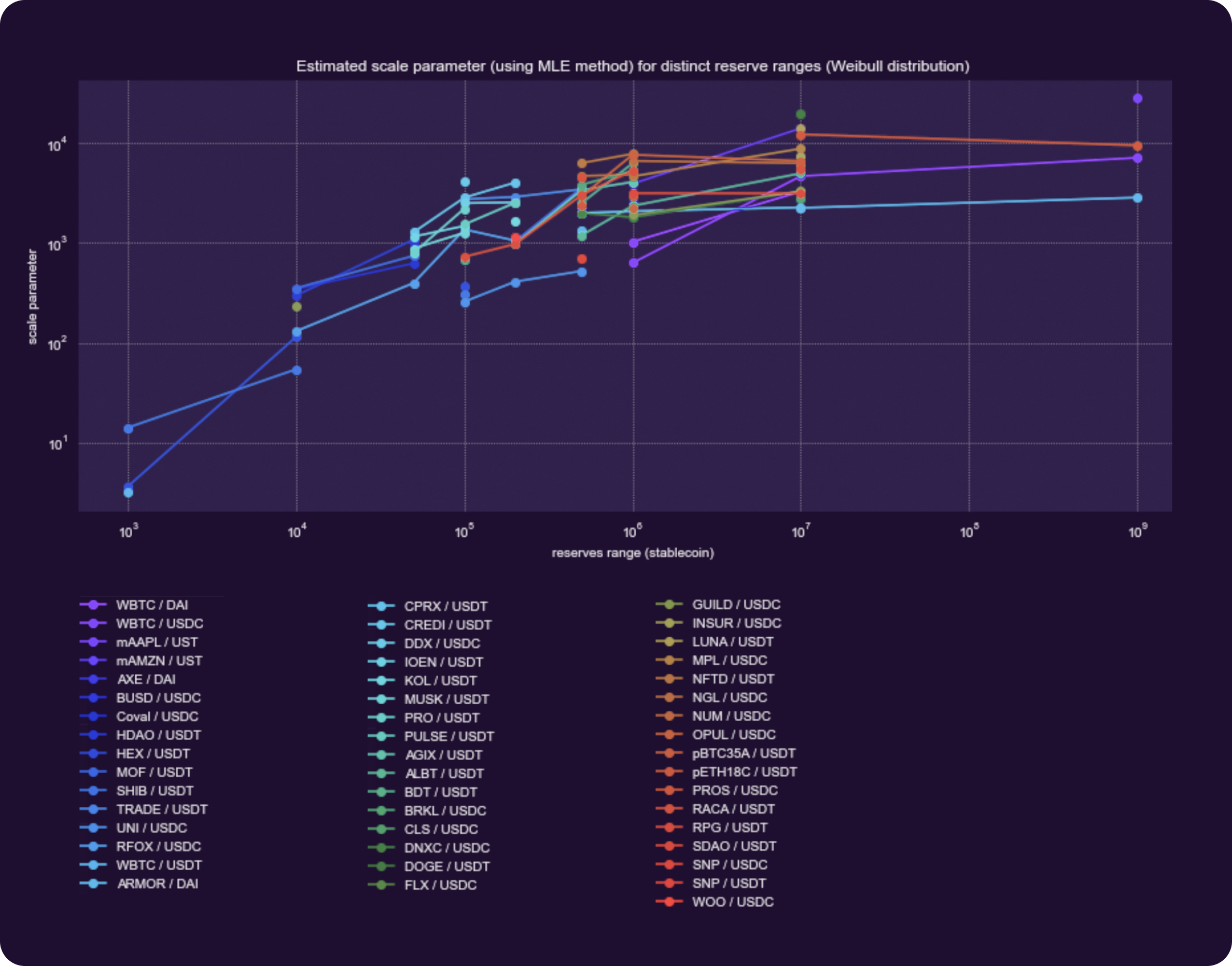Figure 7. Estimated Scale Parameters (using MLE method) for Distinct Reserve Ranges (Weibull Distribution), With X-Axis Representing the Upper Bound Reserve Range

A common trend can be observed — as the range of the reserves increases, the estimated scale parameter also becomes bigger, indicating that for larger pool reserves, the average value of swaps rises.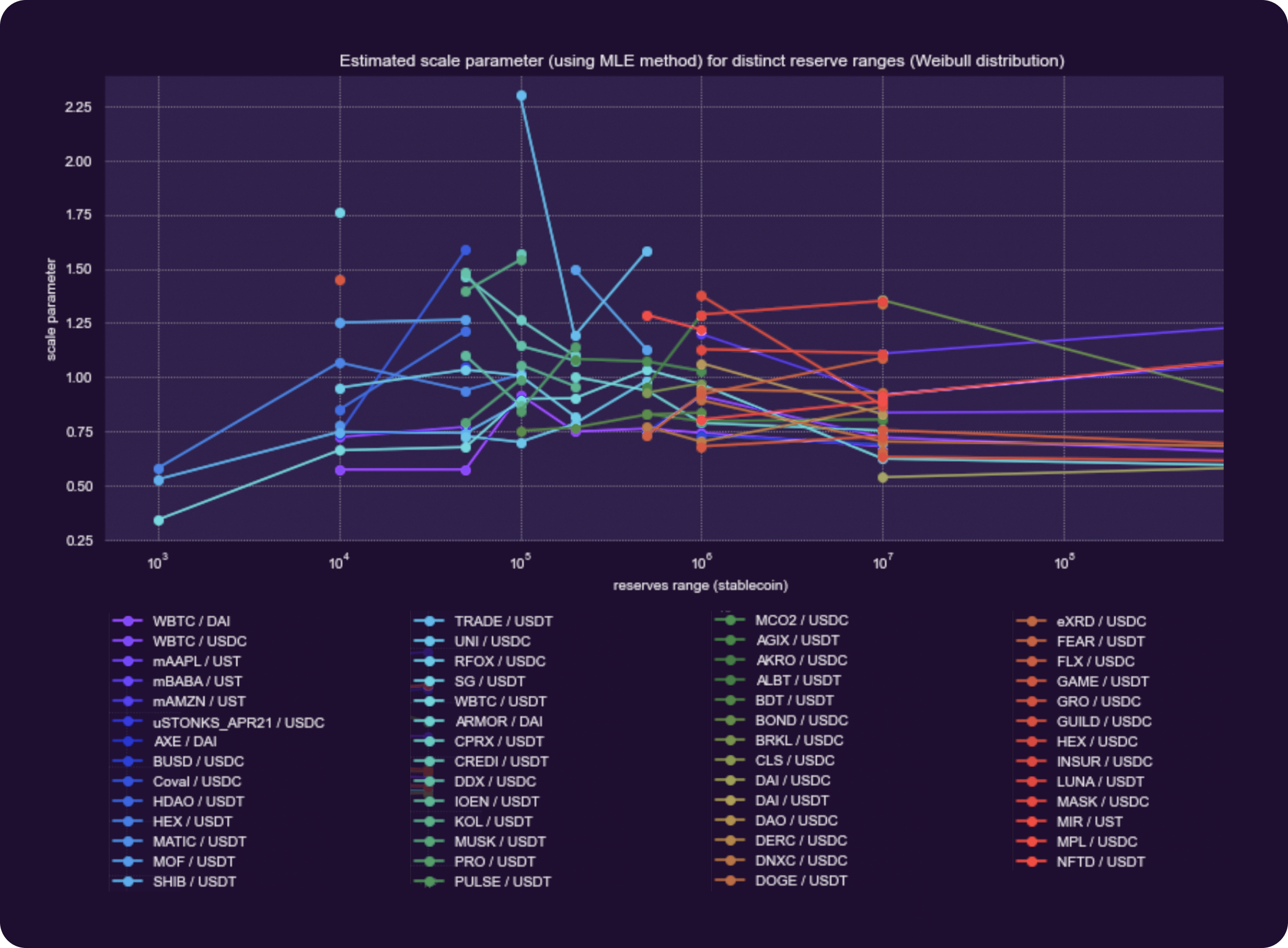Figure 8. Estimated Shape Parameters (using MLE method) for Distinct Reserve Ranges (Weibull Distribution), With the X-Axis Representing the Upper Bound Reserve Range

The shape parameter doesn’t follow a monotonic pattern but from the existing data, it can be observed that it seems to increase toward the middle range of the reserves followed by a consequent smaller decrease till a certain point. The diagram below shows the curves computed by taking the arithmetic mean of the parameters for each range across all of the analyzed pools.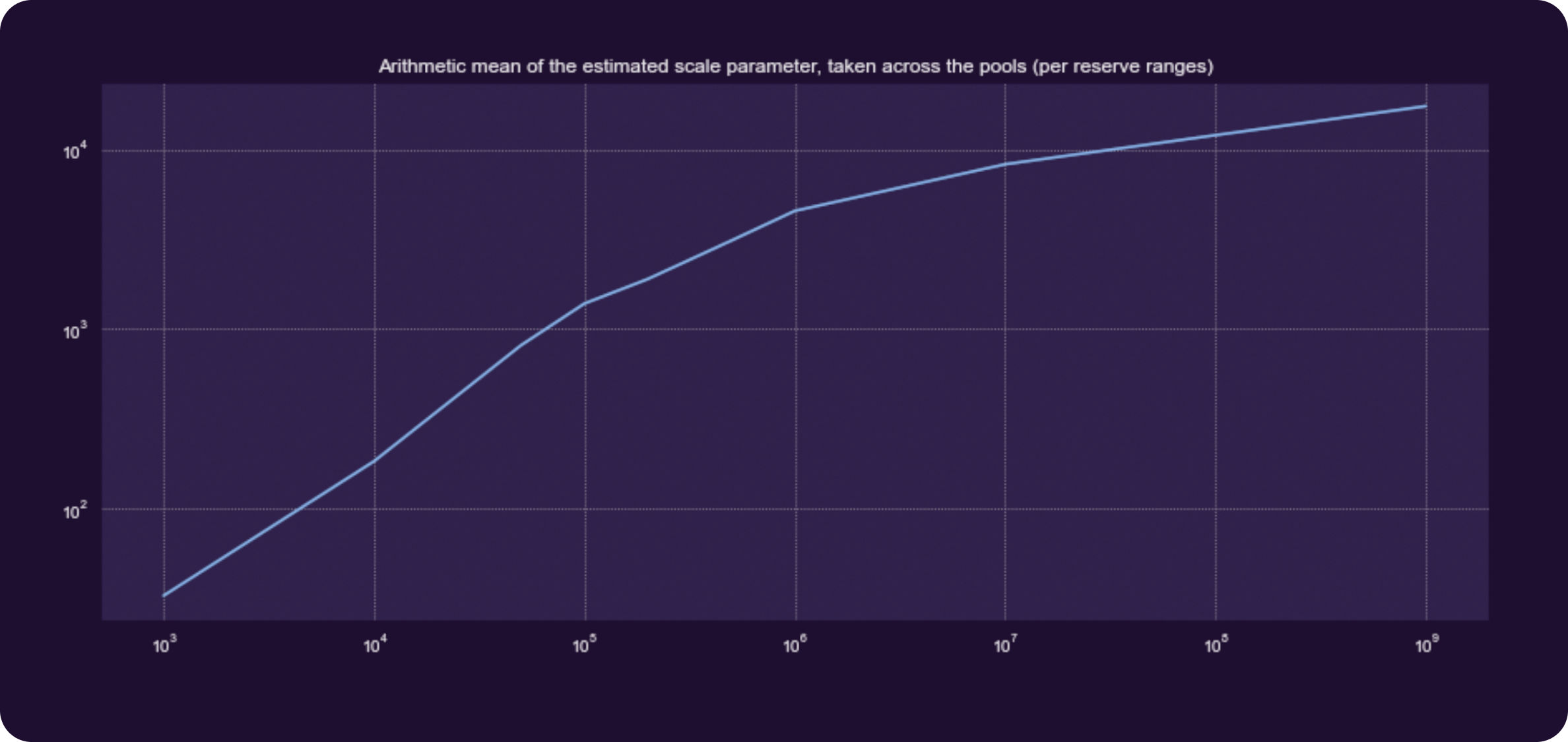Figure 9. Arithmetic Mean of the Estimated Scale Parameter Taken Across the Pools (per reserve ranges), With 200+ Pools Considered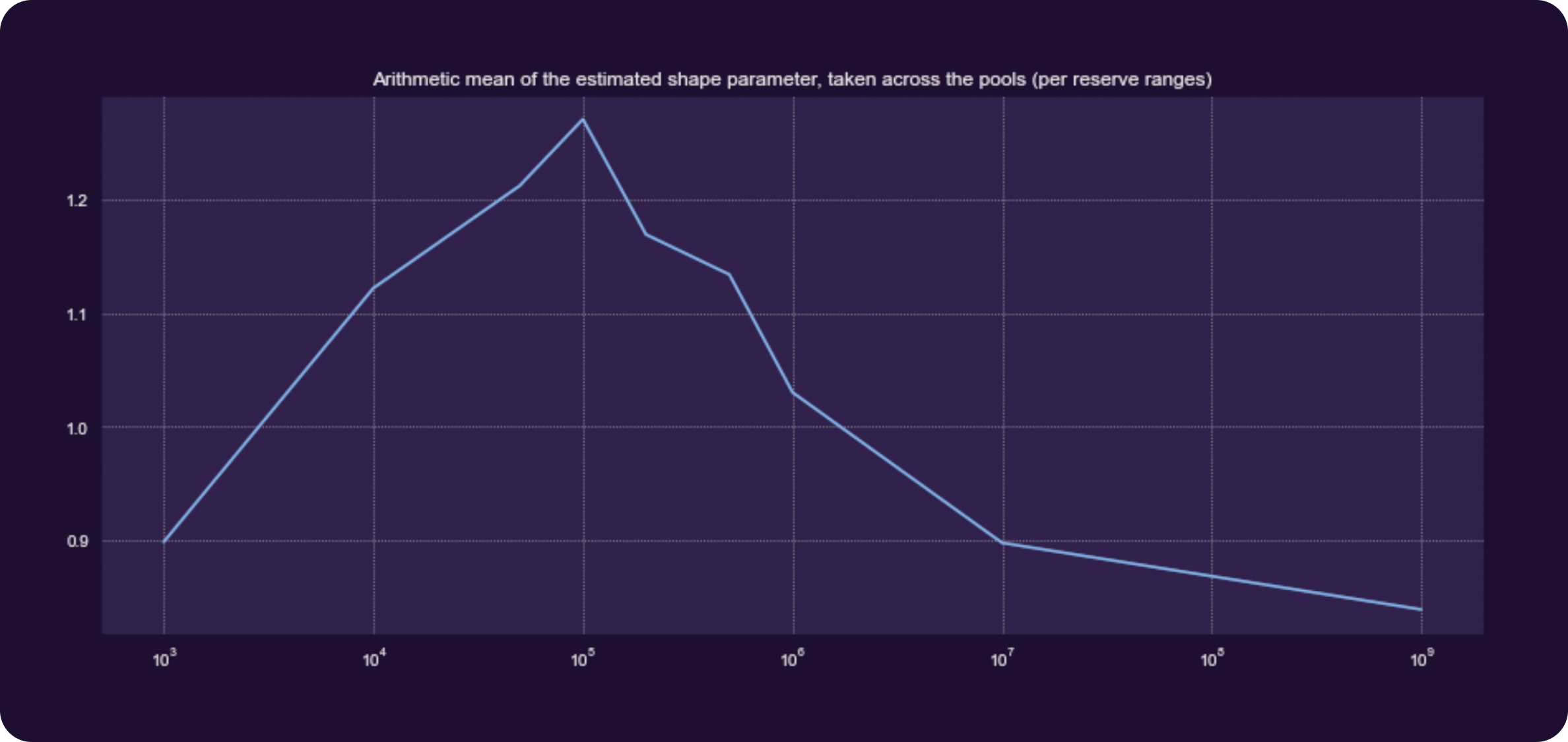Figure 10. Arithmetic Mean of the Estimated Shape Parameter Taken Across the Pool (per reserve ranges) With 200+ Pools Considered

# Generalizing Trade-Size Distribution Parameters

A naive way to generalize the estimated parameters in order to select the ones for conducting the simulations would be to consider the arithmetic mean of scale and shape across distinct pools at each distinct reserve range.

However, this approach doesn’t take into consideration the relationship between the parameters and will certainly not be able to reflect different trading behavior patterns in pools falling inside the same reserve range.

To better understand the point, it’s enough to analyze the relationship between the parameters by constructing a scatterplot of scale/shape values estimated for each pool.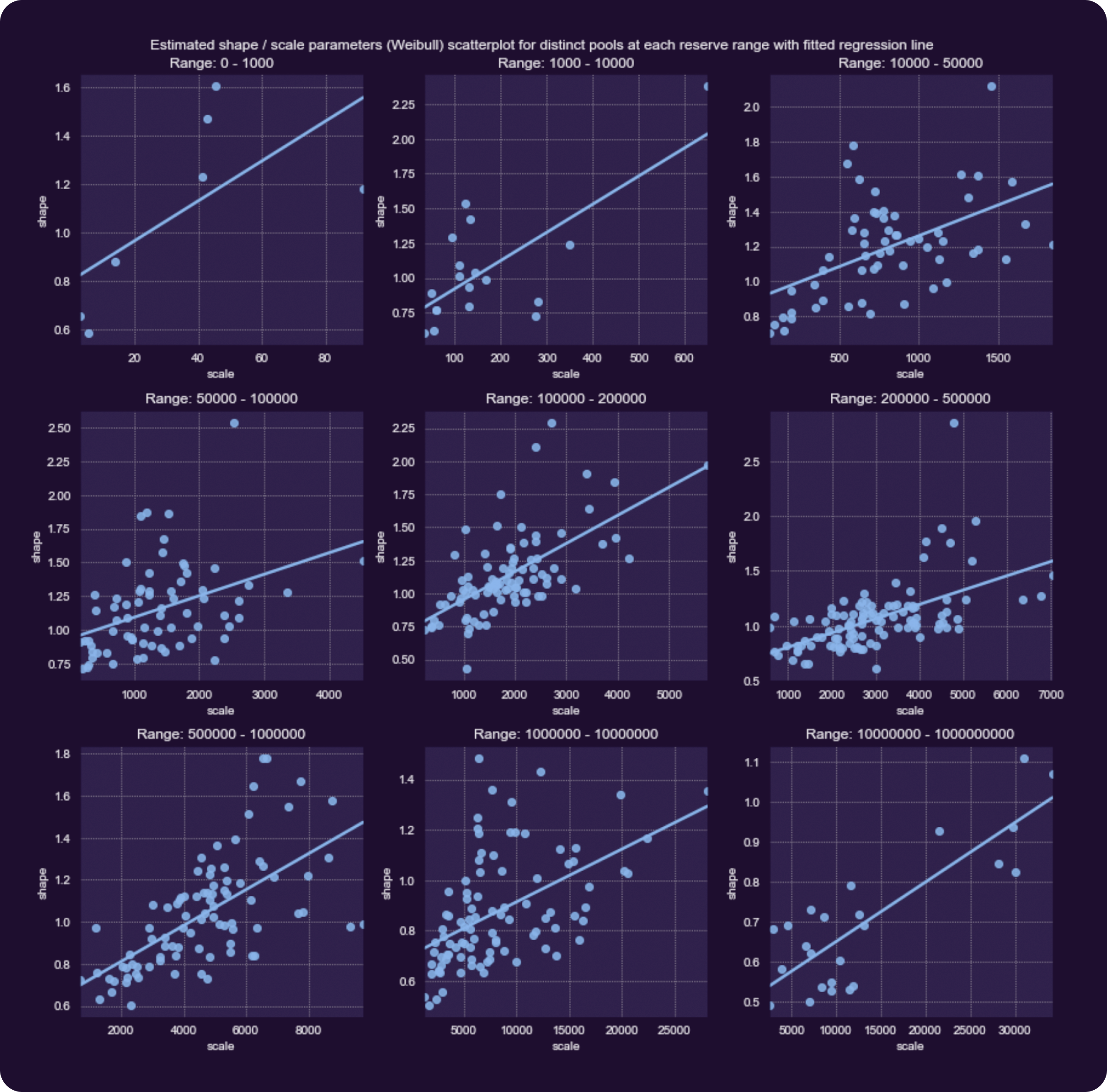Figure 11. Estimated Scale/Shape Parameters (Weibull Distribution) Scatterplot for Distinct Pools at Each Reserve Range With Fitted Regression Line

There is a positive correlation between the shape and scale parameters. It can be explained by taking into consideration the effects of the parameters on the distribution. The scale parameter stretches the distribution to the right, increasing the probability of higher values.

Increasing the shape parameter not only leads to a shift of the distribution mode to the right but also results in a thinner tail, decreasing the probability of extremely high values. By increasing the shape and scale parameters proportionally, the maximum generated values almost don’t vary. Having a high scale value with a low shape, in contrast, results in unrealistically large generated values.

For selecting the final trade-size distribution parameters that would reflect distinct trading behaviours, two (2) methods have been chosen.

The first method consists of computing the four (4) equally spaced points both for the shape and scale parameters, between their minimum and maximum values across pools, and considering all possible pairs. This results in a grid of 4×4 possible combinations. However, this results in a lot of unrealistic scenarios (points in the upper left and lower right corners).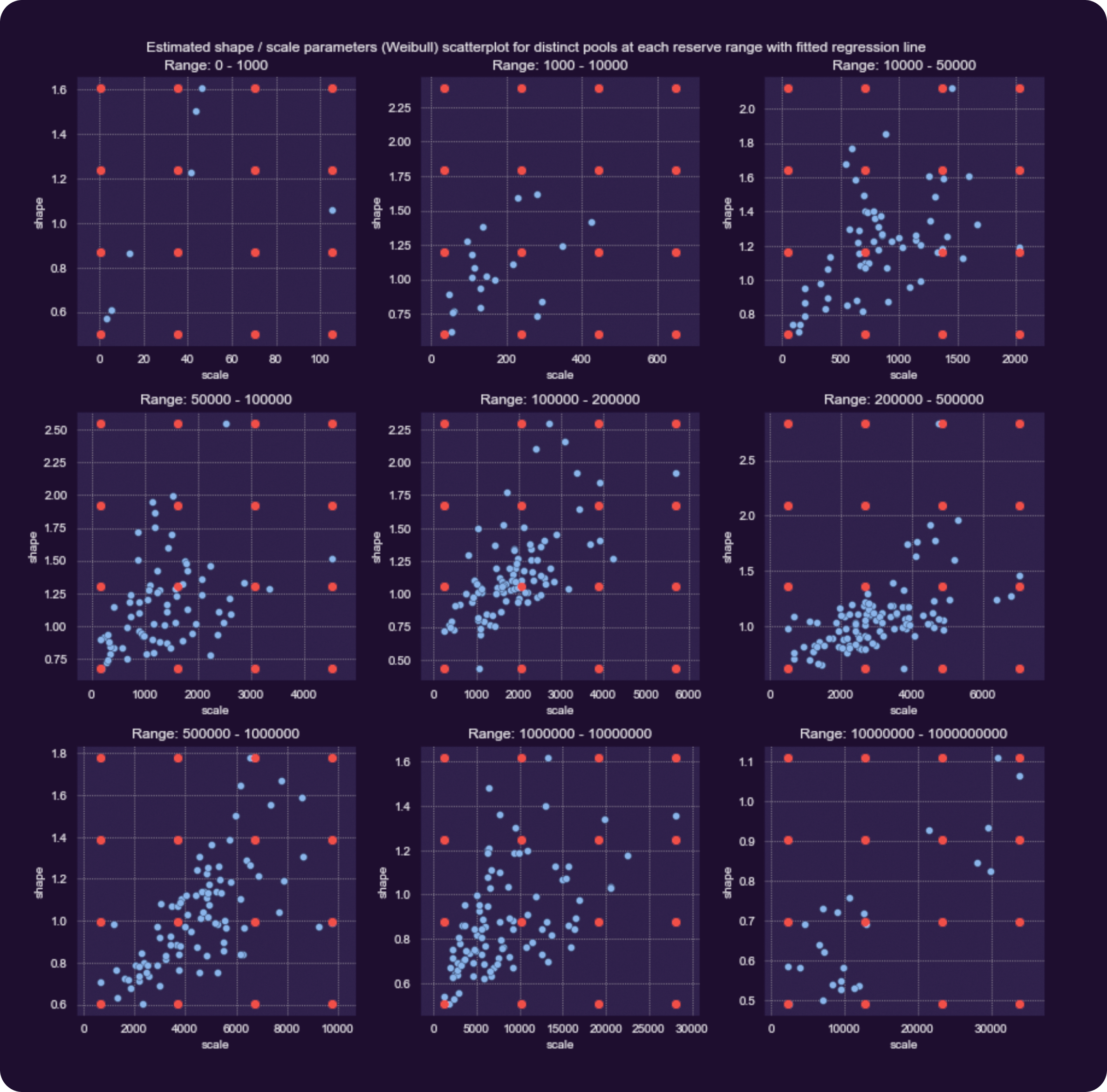Figure 12. Estimated Scale/Shape Parameters (Weibull Distribution) Scatterplot for Distinct Pools, at Each Reserve Range, With Generalized Parameters (Method I) Highlighted in Red

Presented below are the histograms of values sampled from the Weibull distribution, with the parameters corresponding to the highlighted points for the reserve range [50,000-100,000].

By varying the shape and scale parameters, the overall shape of the generated values changes significantly. Each of such combinations describes different patterns in trading behavior. Notice that a smaller shape value not only leads to the shift of the mode to the left but also to a bigger amount of extreme (very large) generated values.

The second method consists of choosing generalized distribution parameters only by considering realistic cases. The same procedure has been applied for each reserved range. Shown below are parameters that have been selected for each range.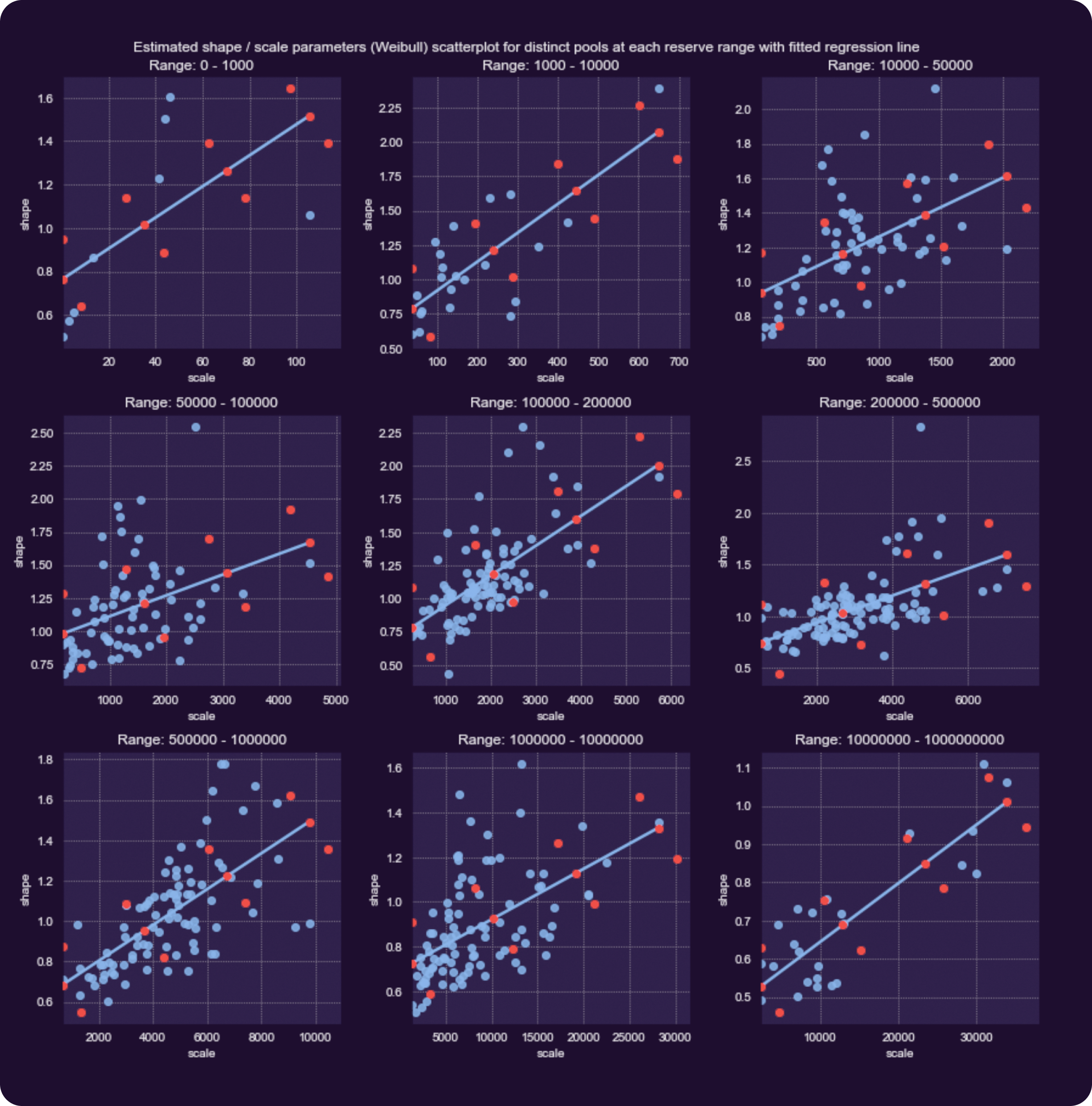Figure 14. Estimated Scale/Shape Parameters (Weibull Distribution) Scatterplot for Distinct Pools, at Each Reserve Range, With Fitted Regression Line and Generalized Parameters (method II) Highlighted in Red

Now, the generalized parameters cover almost the entire range of possibilities. The exact procedure of computing them is described inside the project repository.

# How Often Swaps Are Performed

To obtain realistic values for the transaction frequency generator, it was decided to compute the median daily number of swaps considering the most active 200+ Uniswap V2 pools.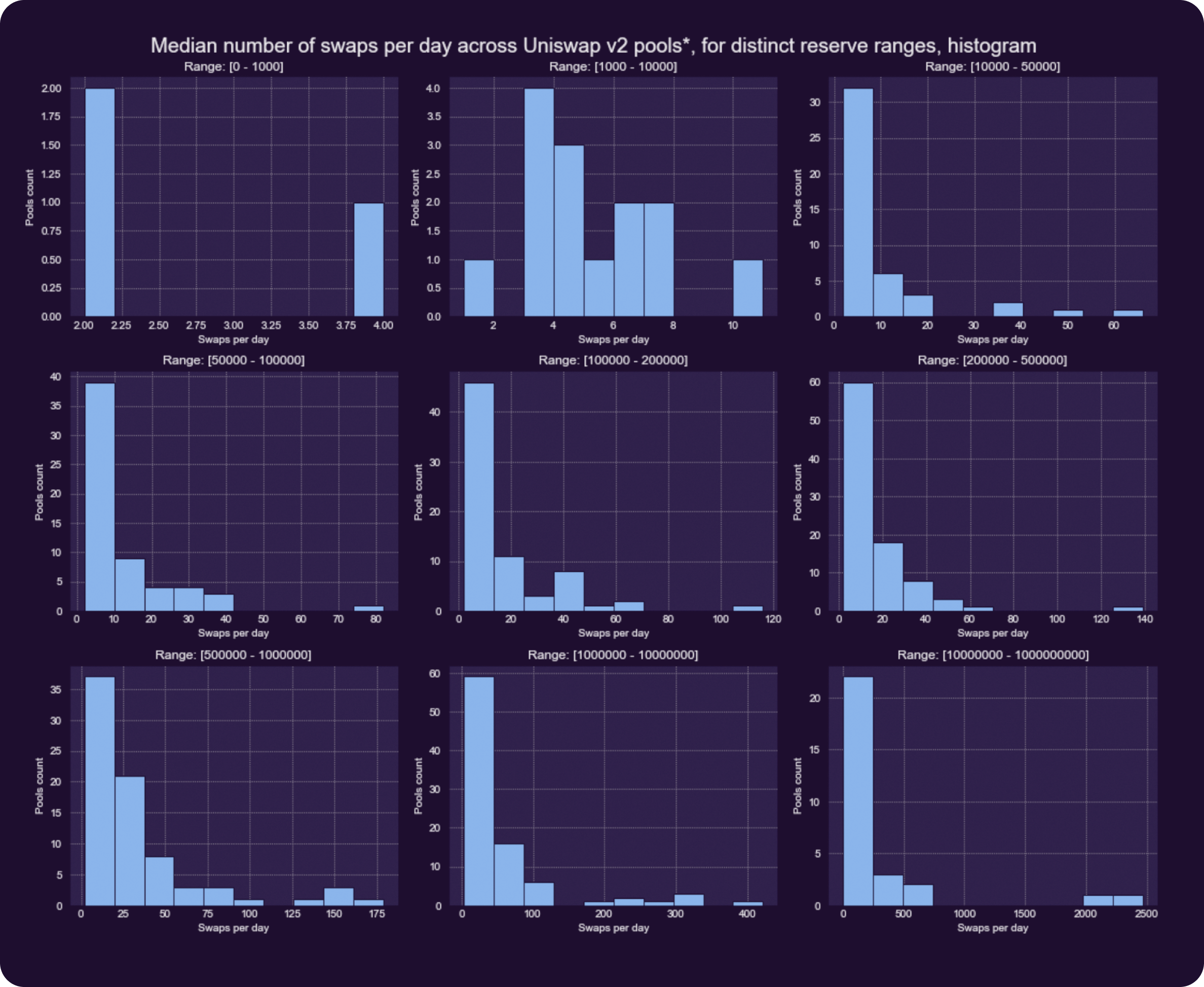Figure 15. Median Number of Swaps per Day Across Uniswap v2 Pools* for Distinct Reserve Ranges, Histogram

A clear pattern can be observed, the greater the pool reserves, the higher the median daily frequency tends to be for the majority of the pools. The highest swap frequency is registered for pools WETH/USDC and WETH/USDT and is greater than 2000 swaps per day. Below, is presented the same histogram capped at 250.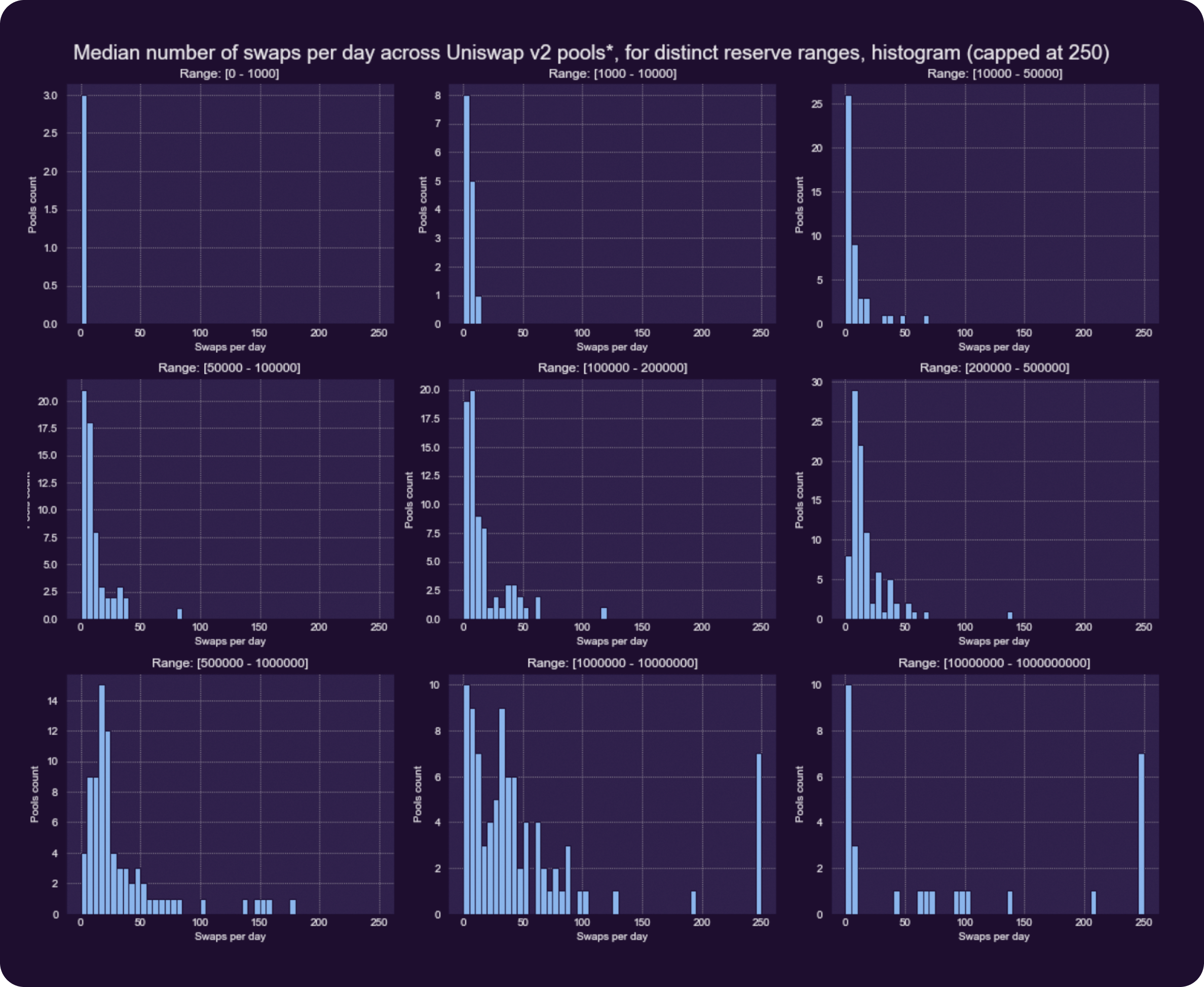Figure 16. Median Number of Swaps Per Day Across Uniswap v2 Pools* for Distinct Reserve Ranges, Histogram (capped at 250)

# Token in Frequency Ratio

During the initial analysis, it was established that the traders’ behavior across pools can differ significantly. In some particular markets, traders tend to perform many small-sized swaps in one direction and rarer but bigger in size transactions (in USD equivalent) in the opposite direction.

Presented below is the histogram of the ratio between the number of swaps on each side computed across the analyzed pools.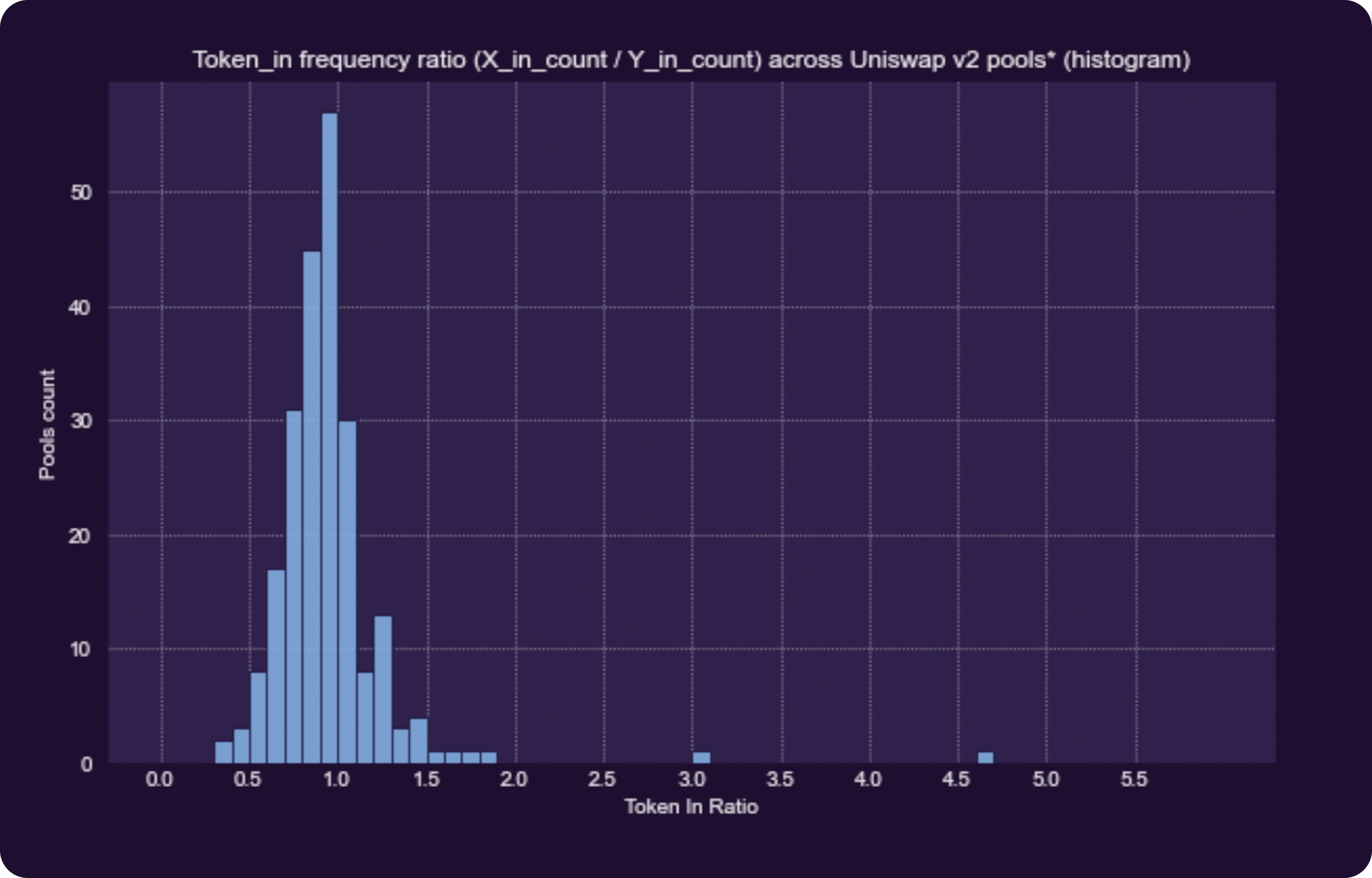Figure 17. Token In Frequency Ratio (X_in_count / Y_in_count) Across Uniswap v2 Pools*, Where Y – Stablecoin, X – the Remaining Token in the Pair

For most pools, the ratio is near 1, meaning that the number of swaps in each direction is the same.

Several extremes can be observed, which were analyzed manually. Particularly, the ratio of about 4.6 corresponds to a token, which happens to be a financial pyramid scheme. After a sudden pump, its price dropped by more than 100 times in a matter of several weeks.

Despite the price decrease, there remains pretty high liquidity of this token inside the pool, and people are still performing mainly swaps in order to exchange the token on the stablecoin, causing such a high token-in ratio.

On the other hand, the pool with a token-in ratio of about 3 corresponds to a healthy pool, where the difference in swap frequency for each side is natural and doesn’t cause significant price variation in the long run (because of the differences in swap sizes, in USD equivalent, for each direction).

The token-in frequency ratio for the majority of the pools falls in the range of 0.5 – 2.

# Conclusion

Mathematical models provide an effective way to describe the behavior of traders and simulate various market situations. By analyzing the historical data, it was possible to identify the best-fit distributions and the parameters specific for distinct pools. Even though traders manifest different behavior in each case, it’s possible to perform a generalization that would describe almost the entire range of possibilities.

By estimating the trade-size distribution parameters, it was shown that the average size of the swaps tends to increase for pools with bigger liquidity. For a given reserve range, in some cases, people mostly perform small-sized swaps, while in others, medium value trades are dominant.

The frequency of swaps also correlates with the pool liquidity. Pools with a high number of daily transactions usually have bigger liquidity. Finally, for a given pool, the behavior of traders for each side may also differ. The exchanges on one side may be smaller in values but more frequent than in the reverse order.

All these insights will allow us to perform realistic market simulations and compare different stress situations.